IF YOU CAN SEE THIS go to /sfa/Nuprl/Shared/Xindentation_hack_doc.html

### Some Type Constructors used to represent Propositions

The following forms of expression were mentioned in Propositions.

P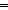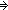Q
P & Q
P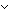Q
False
True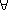x:AP(x)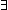x:AP(x)
a = b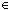T
a<b

As discussed in Propositions as Types we use types to represent propositions. The standard logical symbols are not primitive in Nuprl and we actually use them for the following types and type constructors, which approximate their constructive content. The "weak" correspondence between a type and a proposition consists of the type's having a member just when the proposition is true. The "strong" correspondence between a type and a proposition consists of the type's expressing the computational content of the proposition.

The expression "r = sT" is a primitive that is defined to be a type given that rT and sT. It has a member "*" iff r and s are equal expressions of T. Further,

Thm*r,s:Tx:(r = sT). x = *(r = sT)

The expression "a<b" is treated the same way for a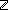, b.

Def Prop == Type

Def PQ == P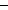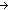Q
Def P & Q == P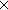Q
Def PQ == P+Q
Def False == Void
Def True == 0Defx:AB(x) == x:AB(x)
Defx:AB(x) == x:AB(x)

A member of "x: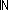y:P(x;y)" is some function in "x:y:P(x;y)".

(Sept 2001 - sfa )